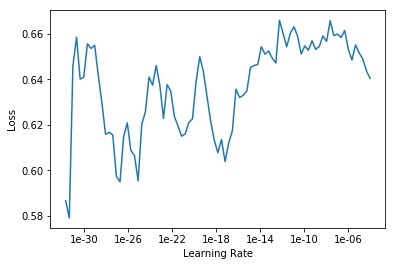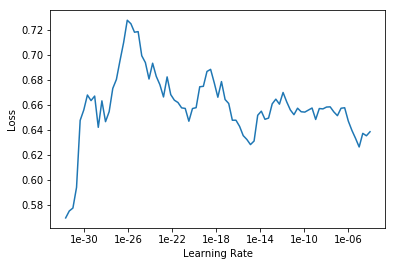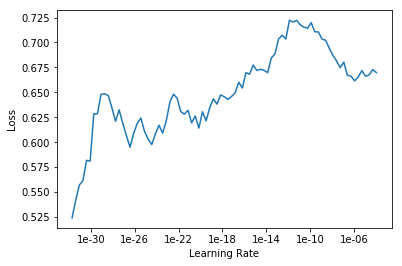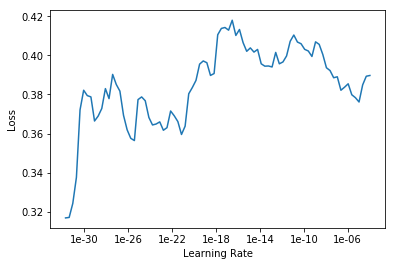# Using lr_find - drastically different plots each time

#1

I am currently on lesson 2, working on an image classifier for cormorants, magpies, blue jays, ravens, crows and cormorants. I’m having a hard time selecting a learning rate because when I run learn.lr_find and learn.recorder_plot I get drastically different plots each time.Each plot was produced on the same ImageDataBunch using the same 4 lines of code:

``````learn = cnn_learner(data, models.resnet50, metrics=error_rate)
learn.fit_one_cycle(1)
learn.lr_find(start_lr=1e-35, end_lr=1e-2)
learn.recorder.plot()
``````

My error rate after fit_one_cycle(1) is usually between 21% and 25% but sometimes as high as 28%) If I do more epochs I can get it down to ~16%-18%, but no better.

Any advice on how I should select the optimal learning rate? Should I run more epochs to get the error rate down to 20% before turning to lr_find?

Note: My “most confused” looks reasonable:
[(‘ravens’, ‘crows’, 24),
(‘crows’, ‘ravens’, 8),
(‘western_jackdaws’, ‘crows’, 6),
(‘crows’, ‘western_jackdaws’, 5),
(‘ravens’, ‘western_jackdaws’, 4),
(‘western_jackdaws’, ‘ravens’, 4),
(‘bb_magpies’, ‘blue_jays’, 2),
(‘bb_magpies’, ‘crows’, 2),
(‘bb_magpies’, ‘ravens’, 2),
(‘crows’, ‘bb_magpies’, 2),
(‘crows’, ‘cormorants’, 2)]

Could the difficulty of differentiating crows from ravens be causing problems?

Note 2:
Could I be creating the DataBunch Incorrectly? When I try to run:

``````interp = ClassificationInterpretation.from_learner(learn)
interp.plot_top_losses
``````

I get:

``````AttributeError                            Traceback (most recent call last)
<ipython-input-55-34fc804e24e7> in <module>
----> 1 interp.plot_top_losses(9, figsize=(15,11))

/opt/conda/envs/fastai/lib/python3.6/site-packages/fastai/vision/learner.py in _cl_int_plot_top_losses(self, k, largest, figsize, heatmap, heatmap_thresh, alpha, cmap, show_text, return_fig)
174     if show_text: fig.suptitle('Prediction/Actual/Loss/Probability', weight='bold', size=14)
175     for i,idx in enumerate(tl_idx):
--> 176         im,cl = self.data.dl(self.ds_type).dataset[idx]
177         cl = int(cl)
178         title = f'{classes[self.pred_class[idx]]}/{classes[cl]} / {self.losses[idx]:.2f} / {self.preds[idx][cl]:.2f}' if show_text else None

/opt/conda/envs/fastai/lib/python3.6/site-packages/fastai/data_block.py in __getitem__(self, idxs)
647     def __getitem__(self,idxs:Union[int,np.ndarray])->'LabelList':
648         "return a single (x, y) if `idxs` is an integer or a new `LabelList` object if `idxs` is a range."
--> 649         idxs = try_int(idxs)
650         if isinstance(idxs, Integral):
651             if self.item is None: x,y = self.x[idxs],self.y[idxs]

/opt/conda/envs/fastai/lib/python3.6/site-packages/fastai/torch_core.py in try_int(o)
365     "Try to convert `o` to int, default to `o` if not possible."
366     # NB: single-item rank-1 array/tensor can be converted to int, but we don't want to do this
--> 367     if isinstance(o, (np.ndarray,Tensor)): return o if o.ndim else int(o)
368     if isinstance(o, collections.Sized) or getattr(o,'__array_interface__',False): return o
369     try: return int(o)

AttributeError: 'Tensor' object has no attribute 'ndim'``````
0 Likes

#2

Note 2:

I create the databunch with:

``````bs=16
np.random.seed(42)
data = ImageDataBunch.from_folder(path, train=".", valid_pct=0.2,
ds_tfms=get_transforms(), size=224, bs=bs, num_workers=4).normalize(imagenet_stats)``````
0 Likes

#3

Note 3: I fixed the AttributeError issue by upgrading PyTorch.

0 Likes

#4

According to the code you posted, you are training the model with the default learning rate of 3e-3 for one (or more) epoch, and then are attempting to find a good learning rate for the frozen model.

``````learn = cnn_learner(data, models.resnet50, metrics=error_rate)
learn.fit_one_cycle(1)
learn.lr_find(start_lr=1e-35, end_lr=1e-2)
learn.recorder.plot()
``````

I think in the lesson 2 the example Jeremy uses the default learning rate for training the model head, unfreezes the entire model using `unfreeze`, and then uses `lr_find` to find a good learning rate for the rest of the training.

To match that example, you will want to train for longer than one epoch, maybe four to six depending on how the metrics are looking, and after unfreezing the model call lr_find. Don’t pass in `start_lr=1e-35`, I have never used a learning rate that low. The defaults for `lr_find` should work in this case.

0 Likes

#5

Isn’t the interpretation of the plot the same for a frozen or unfrozen model (as well as partially trained or untrained)? If I understood the lecture correctly I’m looking for a point on the plot with a consistent downward slope a bit before the loss begins increasing again. That didn’t exist on the plot, so I started expanding the range to include smaller learning rates.

Why are the plots coming out so drastically different each time?

0 Likes

#6

Could it be that I trained the model enough each time that none of the learning rates tested were going to yield improvements consistently, and thus the lr_find plot is essentially noise?

0 Likes

#7

Lesson 2 gives a quick overview of interpreting `lr_find`, I believe Lesson 3 goes into more depth. The short answer is you do look for different areas depending on whether the model has been trained or not.

Part of it is the extreme range of learning rates you are using. You are having it traverse from 1e-30 to 1e-2 in 100 batches (which is the default). Which is a lot of learning rates to test and very little data to test them on.

The other part is `lr_find` isn’t deterministic because there will be differences in augmentations applied, etc. See this post for a longer answer.

3 Likes